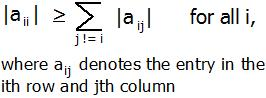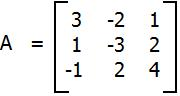Open in App
Not now

# C++ Program for Diagonally Dominant Matrix

• Last Updated : 13 Jul, 2022

In mathematics, a square matrix is said to be diagonally dominant if, for every row of the matrix, the magnitude of the diagonal entry in a row is larger than or equal to the sum of the magnitudes of all the other (non-diagonal) entries in that row. More precisely, the matrix A is diagonally dominant ifFor example, The matrixis diagonally dominant because
|a11| ≥ |a12| + |a13| since |+3| ≥ |-2| + |+1|
|a22| ≥ |a21| + |a23| since |-3| ≥ |+1| + |+2|
|a33| ≥ |a31| + |a32| since |+4| ≥ |-1| + |+2|
Given a matrix A of n rows and n columns. The task is to check whether matrix A is diagonally dominant or not.
Examples :

```Input : A = { { 3, -2, 1 },
{ 1, -3, 2 },
{ -1, 2, 4 } };
Output : YES
Given matrix is diagonally dominant
because absolute value of every diagonal
element is more than sum of absolute values
of corresponding row.

Input : A = { { -2, 2, 1 },
{ 1, 3, 2 },
{ 1, -2, 0 } };
Output : NO```

The idea is to run a loop from i = 0 to n-1 for the number of rows and for each row, run a loop j = 0 to n-1 find the sum of non-diagonal element i.e i != j. And check if the diagonal element is greater than or equal to the sum. If for any row, it is false, then return false or print “No”. Else print “YES”.

Below is the implementation of the approach.

## C++

 `// CPP Program to check whether given matrix` `// is Diagonally Dominant Matrix.` `#include ` `#define N 3` `using` `namespace` `std;`   `// check the given matrix is Diagonally` `// Dominant Matrix or not.` `bool` `isDDM(``int` `m[N][N], ``int` `n)` `{` `    ``// for each row` `    ``for` `(``int` `i = 0; i < n; i++)` `   ``{        `   `        ``// for each column, finding sum of each row.` `        ``int` `sum = 0;` `        ``for` `(``int` `j = 0; j < n; j++)             ` `            ``sum += ``abs``(m[i][j]);        `   `        ``// removing the diagonal element.` `        ``sum -= ``abs``(m[i][i]);`   `        ``// checking if diagonal element is less ` `        ``// than sum of non-diagonal element.` `        ``if` `(``abs``(m[i][i]) < sum) ` `            ``return` `false``; ` `       `  `    ``}`   `    ``return` `true``;` `}`   `// Driven Program` `int` `main()` `{` `    ``int` `n = 3;` `    ``int` `m[N][N] = { { 3, -2, 1 },` `                    ``{ 1, -3, 2 },` `                    ``{ -1, 2, 4 } };`   `    ``(isDDM(m, n)) ? (cout << ``"YES"``) : (cout << ``"NO"``);`   `    ``return` `0;` `}`

Output

`YES`

Time Complexity: O(N2), as nested loops are used
Auxiliary Space: O(1)

Please refer complete article on Diagonally Dominant Matrix for more details!

My Personal Notes arrow_drop_up
Related Articles